## Selecting Records by Criteria

Criteria to select only certain records for the query results is added to the end of the query statement. The IF command along with the Comparison symbols are used to indicate a query condition.

Using the contents of a field as the criteria:

• Using the semi-colon which means does NOT CONTAIN

LIST MST CRS TCH MST.CN MST.SE CRS.CO MST.PD MST.SM IF CRS.CO ; TEACH

• Medical History using a populated code as the criteria

LIST STU SEC MST TCH CRS MHS TCH.TE STU.NM MHS.CD? BY TCH.TE IF MHS.CD > 0

Using Red flags as the criteria:

• Assertive Discipline using the red flag as the criteria

• Flags sorted by table

LIST STU FLG STU.NM STU.GR FLG.TC FLG.CO BY FLG.TC IF FLG.SN > 0

• Flags listed for a specific table

LIST STU FLG STU.NM STU.GR FLG.TC FLG.CO IF FLG.TC = MED

• Flags for a specific student

LIST STU FLG STU.NM STU.GR FLG.TC FLG.CO IF STU.NM : ABBOTT

Using "Null" or " "

• Looking for students with no course requests

LIST STU SSS STU.SN STU.NM STU.GR STU.CU BY STU.CU IF SSS.SN = NULL

• Using a populated user field as the criteria

LIST STU NM PG AD CY ZC TL MW FW IF U7 # " "

Working with dates

• The "?" after BD will produce the age of the student, while the ".MONTH" after BD will return only students born in December

LIST STU TCH TCH.TE STU.NM STU.BD STU.BD? IF STU.BD.MONTH = 12

• Students who have not had the 1st polio shot – no date is entered

LIST STU IMM STU.SN STU.NM IF IMM.P1 = NULL

Using Connectives "AND" and "OR"

• When using AND all records must meet all of the IF conditions.

LIST STU TST CTL CTL.NM TST.SN STU.NM TST.ID TST.GR TST.RS TST.SS TST.PL TST.TD BY STU.NM TST.PT IF TST.ID : SBAC AND TST.TD.YEAR = 2017

• When using an OR the records must meet either condition.

LIST STU GRD CRS TCH TCH.TE STU.NM CRS.CO GRD.M1 IF GRD.M1 : D OR GRD.M1 : F

• When combining AND with multiple OR parts in an IF STATEMENT, encase the OR portion in ( ) and place a space before and after each parenthesis.

LIST STU GRD CRS TCH TCH.TE STU.NM CRS.CO GRD.M1 IF ( GRD.M1 : D OR GRD.M1 : F ) AND STU.GR = 9

NOTE:  The "OR" portion of the condition is placed inside of ( ) so as to be considered one condition when the "AND" portion is added.

# Math Operations

Various math operations can be performed in Query. As usual, there is a space between each command, table, field, operation or condition. You can add, multiply, divide or subtract within the start and stop math symbols.

For example, in the below query the students are listed with their grade and one grade level higher:

LIST STU ID NM GR (( GR + 1 ))The “Class Of” can also be calculated based off of the student’s current grade:

LIST STU ID NM GR (( 2018 + 12 - GR ))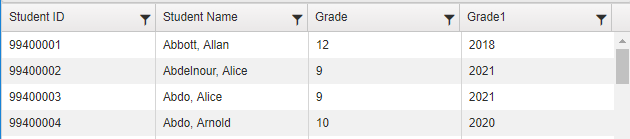In the query below the cumulative credit completed (CC) is being divided by the cumulative credit attempted (CA) and then multiplied (*) by 100 to convert it to a percent:

LIST STU NM CC CA (( CC / CA * 100 )) IF CA # 0In the next example the days present are divided by the days enrolled and then multiplied by 100 to calculate the Attendance Percentage. Formatting is also done to trim the percentages to two significant digits and add a % symbol to the end:

LIST STU NM DE DA DP (( LEFT ( 100 *  DP / DE  , 5 ) + "%" )) BY (( DP / DE )) REV IF DE # 0Changing the 100 to 100.0 in the above query will allow the query to display the calculated value to the hundredths place.This query demonstrates the effects of using the Rounding function:

LIST STU NM DE DA DP (( ROUND ( DP / DE * 100,2 ) + "%" )) (( LEFT (DP / DE * 100,5 ) +"%" )) BY (( DP / DE )) REV IF DE # 0NOTE:  This query does not produce any results when run in Aeries Web Version.  Some Access functions are not supported in Aeries Web Version at this time.

The next example is using the fee amount charged (AM) and subtracting the amount paid (PD) and then only displaying the information if the remaining amount is greater than zero:

LIST STU FEE STU.NM STU.GR  FEE.CD? FEE.AM (( FEE.AM - FEE.PD )) IF (( FEE.AM - FEE.PD )) > 0The outstanding fee balance can also be displayed by inserting the mathematical operation in the field area before the IF. This query could be used for a Fee Letter that would go home to the parents:

LIST STU FEE STU.ID STU.LN STU.FN STU.GR STU.PG STU.AD STU.CY STU.ST STU.ZC (( FEE.AM - FEE.PD )) IF (( FEE.AM - FEE.PD )) > 0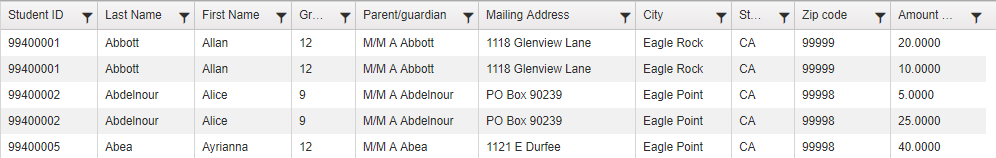Math operations can also be performed to truncate a field or “cut off” characters or numbers from left or right.  The following query examples will truncate to the left all characters up to the first character of a student’s first name:

LIST STU FN (( LEFT ( FN, 1 ) ))LIST STU ID FN LN (( LEFT ( FN , 1 ) + LN ))Another example is truncating the CGPA field left to reduce it to 2 digits.  Take note to the truncation (CP,3).  The reason for this is so that it will include the decimal point:

LIST STU CP (( LEFT (CP, 3) )) IF CP > 0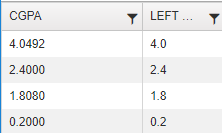In the following query the birth date is truncated to only include the last four digits and will only display the birth year:

LIST STU NM BD (( RIGHT ( BD , 4 ) ))The next two examples will use the MID function to list a certain number of characters within a field:

LIST TCH TE (( MID (TE,8,30) )) IF TE : "ath dir "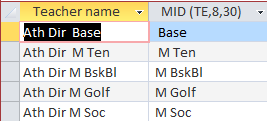LIST CRS CN (( MID (CN,3,4) ))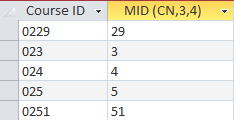The next query demonstrates how to change a whole number to an integer using the INT function:

LIST STU ID NM TP (( INT(TP) )) (( INT(TP*100) ))The following queries using an IIF function to only report information that returns a value based on another value that is being returned. In the first query the word “Boy” will be returned if the student’s sex is M, otherwise “Girl” will be returned.

LIST STU ID NM GR SX (( IIF( SX = "M","Boy","Girl") ))In this example the phrase “Doing Well” will display if the student’s cumulative weighted GPA is > or = 3.000, display “Need to do more"  if the GPA is > 2, and display “At Risk” if the GPA is <  or = 2.000:

LIST STU ID NM GR TP (( IIF(TP>=3,"Doing Well", IIF(TP>2,"Need to do more","At Risk" ) ) ))NOTE:  The MID, INT and IIF functions are not compatible in Aeries Web Version.

In the next example the dash in a student’s last name is removed in the query results by using the Replace function:

LIST STU (( REPLACE(LN,"-","") )) LN FN IF LN : "-"Math Operations can also concatenate or combine fields and strings.  For example, the student’s last name and first name can be joined.  In the example below the students last name is joined with the first name and has a comma inserted between them.  But take note to the first selection after STU, which is NM.  Notice that NM is nowhere listed in the STU Fields.   Aeries Query NM is a programmed concatenation of a student’s last name, plus comma, space and last name.

LIST STU NM (( LN + "," + FN ))Math Operations can also perform operations, concatenate and truncate with in the same query.  The math operation below has calculated from days present (DP) and days enrolled (DE) the actual percentage that a student has been present.

LIST STU NM DE DA DP (( LEFT ( 100 *  DP / DE  , 5 ) + "%" )) BY (( DP / DE )) REV IF DE # 0